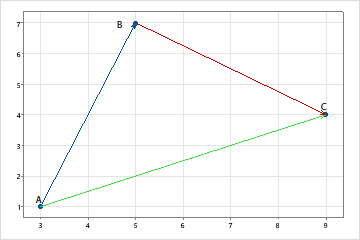# Overview for Pairwise Slopes

Use Pairwise Slopes to calculate the slopes between two columns when each row represents a coordinate point on the X-Y plane. For example, the following data contain three coordinate points, which are shown on the scatterplot: (3, 1), (5, 7), and (9, 4). The pairwise slopes for these data are the slopes of the straight lines between each pair of coordinates.
X Y
Point A 3 1
Point B 5 7
Point C 9 4Slope AB = (7 – 1) / (5 – 3) = 3 Slope AC = (4 – 1) / (9 – 3) = 1/2 Slope BC = (4 – 7) / (9 – 5) = −3/4

## Where to find this analysis

To perform pairwise slopes, choose Stat > Nonparametrics > Pairwise Slopes.